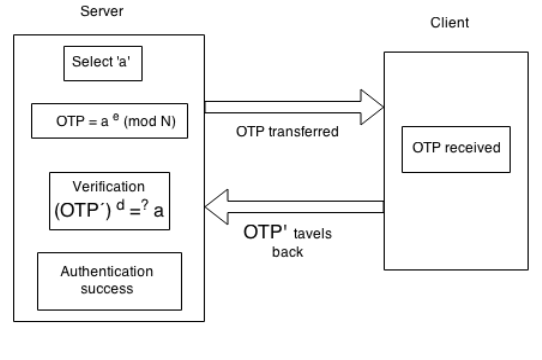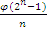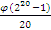Disclaimer: This is an example of a student written essay.

Any opinions, findings, conclusions or recommendations expressed in this material are those of the authors and do not necessarily reflect the views of UKEssays.com.

# An RSA-Type OTP Generator

 ✅ Paper Type: Free Essay ✅ Subject: Computer Science ✅ Wordcount: 2897 words ✅ Published: 12th Mar 2018

An RSA-Type OTP Generator

• Aiswarya Vinayachandran, Sivasankar M

Abstract

Simple and secure authentication protocols are in great demand due to the ever expanding use of internet for financial and message communications. Multifactor authentication, in particular 2Factor Authentication (2FA) is preferred to static passwords-only authentication. One Time Passwords (OTPs) play a vital role in the construction of 2FA protocols. In this paper, an efficient OTP generation algorithm, based on RSA scheme is discussed. Implementation and computational issues related to the algorithm are also discussed.

Keywords: Authentication, RSA, One Time Password, LFSR, Primitive Element

1. Introduction

These days, almost all our day to day activities, starting from buying vegetables to booking a movie ticket depend on internet. As highly private data is being communicated between the server and the client, secure protocols are required for protecting these transactions from attackers. Over the years, we realized that encryption methods alone are not sufficient to secure online transactions. Hence evolved the idea of sending some message each time personally to the user and prompting him to send back the message along with his/her password to complete the transaction. This provides a second layer of security and strength to the existing concept of static passwords.

If you need assistance with writing your essay, our professional essay writing service is here to help!

In this paper, we present a way to generate OTPs, based on RSA type exponentiation. This research paper is organised as: Section 2 explains authentication process; Section 3 briefly discusses the conventional way of OTP generation; Section 4 is the proposed algorithm; Section 5 discusses about the randomness in the generation of the OTPs; Section 6 analyses the operational complexity and security of the proposed algorithm; Section 7 gives some concluding remarks.

2. Authentication

Authentication is the process of identifying the legitimate user . The identity is proven by various cryptographic methods where the user has to enter some input to the system. This can range from simply entering a password to more complicated security mechanisms like biometrics, strings displayed by tokens, key encryptions. Based on this input, the system will identify and authenticate the person. After authentication, comes authorization, where the system identifies the various privileges available to the user. Only authorized users can get access to the data as not all the users will have the same privileges. Some users will be allowed to only read the data while some users will be allowed to read as well as modify it.

2.1. Message Authentication

Message authentication is used to check if the received message has been tampered in the middle of the communication channel. Message authentication is used to protect the integrity of the message wherein the receiver should be notified if any bits in the message are modified, removed or extra bits are added during the communication. This is achieved by sending a message digest – usually hash of the message will be the digest – together with the message. If the receiver also is obtaining the same digest over the received message then he/she can be sure of the integrity of the message.

2.2. Entity Authentication

Entity authentication is the process in which an entity (machine/human) in a distributed network will get belief on another entity (machine/human) based on a key already established between them. The idea is that the key is kept secret and only the two genuine communicating entities know the secret key.

Machine authentication is achieved through the verification of digital credentials or digital certificates. Digital Credentials are like a machine provided ID and password or a digital certificated issued by a Certifying Authority (CA). It is like a digital passport that provides trusted identification. Digital Signature is a mathematical technique used to validate the authenticity of a digital document, software or a message. It is used to identify whether a communication is impersonalized.

Human based authentication relies on at least one of the three key factors: something the user knows (a password or an answer to a security question), something the user possesses (an object for authentication, say smart card), and something the user is (behavioural or physiological characteristics of the individual say, finger print and retina scanning).

3. Conventional OTP Generators

OTP is an authentication technique, which comes in the second layer of authentication protocols after static passwords. An OTP is valid only for a single transaction. Even if an attacker succeeds in decrypting the password of a user, he/she has to get the OTP generated to validate the transaction. Since OTP is based on randomness/collision resistance, it is very difficult to guess an OTP. Even if the attacker succeeds in acquiring an OTP, he may not be able to predict the next OTP.

OTP generation is based on hashing algorithms. Hashing is an irreversible process, i.e. for an input we can get the output, but with the obtained output we cannot get back the input. Even if an attacker obtains many OTPs, it is of no use as he/she cannot find a pattern to guess the seed used to generate the OTPs. An OTP is valid for a limited time, generally two to fifteen minutes based on the web site’s restrictions. Also in online transactions, while entering an OTP, a user is allowed to make errors only a limited number of times, say twice or thrice, which again adds to its security.

A most common way of generating a sequence of OTPs is described in Algorithm 1.

 AlgorithmOTP(seed) Input : a random 20 bit seed Output : a 6 digit OTP 1. A hashing function f is selected 2. f1=f (seed) f2=f (f (seed) =f (f1) = f2(seed) . . . fn=f (f … f (seed))=fn(seed) 3. Initialise i to 0 4. The last value, fn is stored in the server and fn-1 is sent to the user as OTP 5. When the user type fn-1in the transaction site, upon receiving, the server hashes it and compares it with the value stored in the server 6. If the value matches, the user is validated as a genuine user and he/she will be able to proceed with the transaction. 7. If the value doesn’t match, n=n-1,i=i+1 and if i<3 and go to step 6.

Algorithm 1: Conventional OTP Generation Algorithm

Note that the weakness of the OTP mechanism lies on the channel used to send the OTP and the security of the device to which the OTP is send. It will be advisable to secure the device with some biometric credentials making it totally safe.

4 Proposed RSA type OTP Generating Algorithm

After the invention of public key cryptography, encrypted communication reached the next level. In general, public key cryptography relies on some hard mathematical problems like Integer Factorisation Problem (IFP), Discrete Logarithm Problem (DLP) .

As our proposed OTP generation is based on RSA crypto-system, we briefly do a recap of RSA encryption .

4.1 The RSA Algorithm

The Rivest-Shamir-Adleman (RSA) algorithm is one of the popular and secure publickey encryption methods. The security of the algorithm relies on the fact that there is no efficient way to factor very large numbers. Using an encryption key (e, N), the algorithm is as follows:

1. Choose two very large prime numbers, p and q; Set N equal to p.q.
2. Choose any large integer, d, such that gcd(d, ï¦(N) ) = 1.
3. Find e such that e.d = 1 (mod ï¦(N)); The encryption key (e,n) is made public. The decryption key d is kept private by the user.
4. Represent the message as an integer between 0 and (N-1).
5. Encrypt the message by raising it to the eth power mod n. The result is the cipher text C.
6. To decrypt the cipher text message C, raise it to the power d mod n

4.2 Proposed OTP Generation Technique:

Our proposed algorithm is based on RSA encryption/decryption process and is described in Algorithm 2 below.

 Setup 1. Select a large RSA number, N = pq,p and q are two prime numbers 2. Select a number e ïƒŽ {1, 2, 3,… ,ï¦(N) – 1} such that gcd(e, ï¦ (N)) = 1. 3. Find d, the inverse of e such that ed ≡ 1 mod(ï¦(N) OTP Generation 1. Take any random number a ïƒŽ ZN* 2. OTP = ae (mod N) OTP Verification 1. Upon receiving OTP’,if (OTP’)d = a, then authentication is a success.

Algorithm 2: Proposed Algorithm

The above procedure can be represented by a schematic diagram as in Fig.1.Fig. 1. Architecture of the Proposed Model

4.3. A Comment on the Selection of N and the Possible Number of OTPs

Present day OTPs are of generally 6 digits in length. Hence they can range from 000000 to 999999, totalling to 10,00,000. This is so, as we have 10 choices (numbers 0 to 9) for every digit and hence 10.10.10.10.10.10 = 106 = 10,00,000. If we incorporate a module to condition that the first two most significant digits should be non zero, even then 9.9.10.10.10.10 = 8,10,000 OTPs are available. In our proposed algorithm, if we require 6 digit OTPs, we can select N close to the integer 999999. For example a choice of 991 . 997 = 988027 will be sufficient for our implementation. As the number of bits used to represent a 6 digit decimal number is approximately 20 bits (log2 999999 =19.93156713), we need to select a 20 bit RSA number for our algorithm. Note that, a 20 bit RSA crypto system can be easily broken by the present day computers when e and N are known outside. But here as the attacker does not know N and a, he/she cannot guess the next OTP, which is some random number that lies between 1 and N-1.The only information that the attacker can get is the current OTP, which is some 6 digit number.

5. Randomness in the Generation of the OTPs from ZN*

Considering the demand for OTPs and the computational expenses of different exponential algorithms, it is advisable to follow a systematic approach for the selection of the random number aïƒŽ {1, 2,… ,N–1} .We propose two convincing methods for the selection of a.

5.1. Linear Feedback Shift Registers (LFSRs):

LFSR is a mechanism for generating random numbers based on the initial seed given to it. So if we start with a non-zero 20 bit string, the LFSR can generate all the other 220–1 20-bit strings. We refer to  for some basic facts about LFSR.

An LFSR of length L consists of L stages 0,1 , … , L-1, each capable of storing one bit and having one input and output and a clock which controls the movement of data. During each unit of time the following operations are performed; (i) the content of stage 0 is output and forms part of the output sequence; (ii) the content of stage i is moved to stage i – 1 for each i, 1 ≤ i ≤ L – 1; (iii) the new content of stage L – 1 is the feedback bit s which is calculated by adding together modulo 2 the previous contents of a fixed subset of stages 0,1, … , L – 1.

We note that for an n-bit LFSRconnectionpolynomials are available, where ï¦ is the Euler’s totient function. So, for a six digit OTP, i.e. for a 20 bit string, we have= 24,000 choices. With each connection polynomial, we can generate all the 20-bit strings in different random ways. Since we have 24,000choices, we can assign a single connection polynomial for a single customer, and OTPs generated for each customer will be in entirely different pattern.

5.2 Primitive Roots:

Another mechanism for generating 6 digit random numbers is by using the concept of primitive roots.We refer  for the concepts related to cyclic groups and generators/primitive elements..Let p be a prime number. Consider Zp*. Let gïƒŽ ZP*. As i vary from 0 to p–1, by computing gi mod p, we can generate all elements in Zp*. Here g is called the primitive root/generator of Zp*.

Our academic experts are ready and waiting to assist with any writing project you may have. From simple essay plans, through to full dissertations, you can guarantee we have a service perfectly matched to your needs.

As we have selected an RSA number N, which is not a prime number, to follow this kind of random number generation, we can N as a prime number very close to 999999. For example, N = p = 999983 will be sufficient. It is a known result that, if g is a primitive root, then gi is also a primitive root if gcd (i,ï¦(p))=1. Hence we are available with ï¦(ï¦(p)) generators . Hence if N = 999983 (a six digit prime), we have ï¦(ï¦(999983)) =493584 generators which means we have sufficiently large number of primitive roots at our disposal.

6. Computational Complexity and Security of the Proposed Algorithm

The proposed algorithm is an RSA-type algorithm which uses modular exponentiation for its computation. The modular exponentiation operation generally consumes a considerable amount of time for large operands as it consists of a series of square-and-multiply operations under a modular value. For a particular user, e will be fixed. Hence the time complexity for ae (mod N) is O(log2e). As RSA is a widely implemented cryptosystem, improvements in modular exponentiation algorithms are evolving very frequently . Though the proposed algorithm uses the concept of RSA with a 20-bit modular (where as the current standard is 256 to 512 bits), since a, e, N are not known publicly we achieve security through obscurity.

7. Conclusions and Future Works

In this paper, we have proposed a new method to generate OTPs and discussed the possible ways of implementing it practically. There may exist other novel methods with less time complexity. Incorporating new methods we can design more efficient algorithm for generating OTPs. The possibility of generating alphanumeric OTPs will be also explored, in future.

References

Bruce Schneier, “Applied Cryptography“, Wiley Publications, 2002.

 L. Lamport, “Password authentication with insecure communication,” Communications

of the ACM,vol.24,no.11, pp.770-772,1981.

 Neal Koblitz, “Towards a Quarter Century of Public Key Cryptography”, A Special Issue of

Designs, codes and Cryptography, Vol. 19, No. 2/3, Springer, 2000.

 Rivest R. L. ,Shamir A.,Adleman L., “A Method for Obtaining Digital Signatures and

Public-key Cryptosystems”, Communications of the ACM, Vol. 21, No. 2, pp. 120-126, 1978.

 Alfred, J., Van Menezes Paul, C., Oorschot, S., Vanstone, A. “Handbook of Applied

Cryptography” , CRC Press LCC (1996)

 James K Strayer, “Elementary Number Theory” ,Waveland Press, 2001.

 Gueron, Shay and Krasnov, Vlad , “ Software Implementation of Modular Exponentiation, Using Advanced Vector Instructions Architectures” , LNCS Vol. 7369, pp.119-135, Springer, 2012.

View all

## DMCA / Removal Request

If you are the original writer of this essay and no longer wish to have your work published on UKEssays.com then please:

Related Services

Prices from

\$USD152

Approximate costs for:

• 1000 words
• 7 day deliveryHumanity University

Dedicated to your worth and value as a human being!

Related Lectures